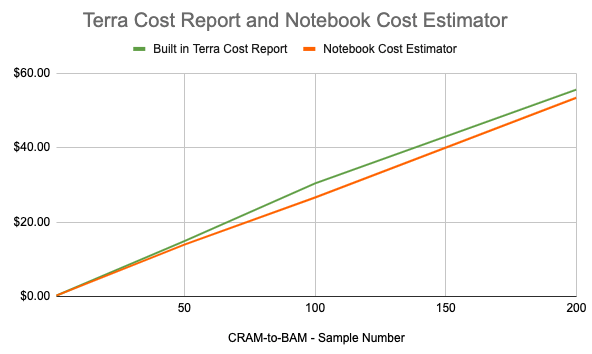# Cost estimating notebook (deprecated)Allie Cliffe
• Updated

If you need granular (task-level) cost reporting, or costs of running workflows, you can estimate the cost of running your workflow with a Workflow Cost Estimator notebook. Follow the instructions below to find the workflow cost estimating python code to run in a notebook. Note that this is currently broken and not supported. However, it is offered as an example of a possible alternative approach for those comfortable with coding.

## Cost-estimate notebook overview

#### Notebooks allow cost reporting for workflows in progress

You can run the cost-estimating notebook at any point in the workflow execution and it will list how much the workflow has cost thus far. You can use as an early indicator of whether you may exceed your budget.

#### Notebooks give more granular cost details

Unlike Terra's cost report, which only provides the total job cost, the notebook estimates task-level and instance-level costs (i.e. the cost of running a particular task within a workflow). This is useful when you want to know what task(s) are responsible for most of the cost.

The notebook gives an estimate; built-in reporting is a final cost. However (see below), this estimate is usually fairly accurate.

Originally created for BioData Catalyst Powered by Terra, an archived version is available on github at https://github.com/DataBiosphere/featured-notebooks/tree/master/attic workspace. You will need the Workflow Cost Estimator notebook.

The archived version includes the python code, which you can run in a notebook in Terra. Note that this notebook is broken (deprecated) and no longer supported. It is intended as an example of a notebook approach only.

### What to expect

The notebook uses FireCloud Service Selector (FISS) to request information on all the submitted jobs associated with the workspace.

The notebook will list all the submission IDs on the screen, and you'll choose which submission to process for cost estimates. The notebook will then use FISS to obtain metadata information about the particular submission - such as how many VMs were used, the number of CPUs used, the duration for each VM, etc. A cost formula uses this information to calculate the cost of running the workflow. The cost formula is based on the GCP's price estimate per resource.

### How accurate are notebook-generated workflow cost estimates?

These cost estimates do not come directly from Google billing. Instead, the notebook calculates a cost estimate based on metadata from Terra. The estimates are (usually) very close to the real cost, though they could be slightly off. Below are descriptions of what's accounted for in the calculations, and the difference between the notebook results, and what Terra's built-in results showed in a benchmark.

### Included GCP costs

Note that estimates will be lower than actual costs, because the notebook cost formula does not account for all possible GCP resources. The table below lists each parameter available in a WDL runtime block (i.e. what type of GCP resource is used for a task) and whether it's included in the cost formula.

 WDL Runtime Parameters Accounted for in Formula? CPU/GPU* Yes Memory Yes Preemptibles Yes Disk Yes Data Egress No noAddress (rarely used) No cpuPlatform (rarely used) No zones (rarely used) No

* Currently, the cost formula assumes all instances are of type N1 listed here, which uses the least expensive type of CPU instance - even if GPUs are being used in the workflow execution.

## Benchmark: Terra cost report versus notebook cost estimates

The two spend report options were benchmarked by running the Cram-to-Bam workflow N times on different CRAM samples. The notebook cost-estimates and built-in cost reporting showed an average difference of \$0.02 per sample/run.

Note that when running larger sample sets, there will be a larger differences as minor differences accumulate.

 CRAM_to_BAM - Sample Number Terra Cost Report Notebook Cost Estimator 1 \$0.22 \$0.23 50 \$14.87 \$13.95 100 \$30.39 \$26.59 200 \$55.62 \$53.41